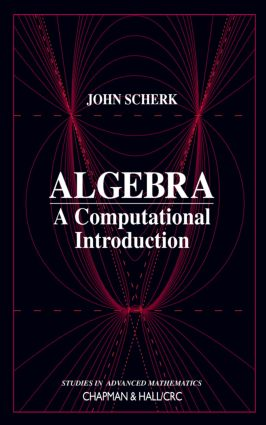Algebra

A Computational Introduction, 1st Edition

Chapman and Hall/CRC

336 pages

Purchasing Options:\$ = USD
Hardback: 9781584880646
pub: 2000-06-23
SAVE ~\$27.00
\$135.00
\$108.00
x
eBook (VitalSource) : 9781315273167
pub: 2018-10-03
from \$67.50

FREE Standard Shipping!

Description

Adequate texts that introduce the concepts of abstract algebra are plentiful. None, however, are more suited to those needing a mathematical background for careers in engineering, computer science, the physical sciences, industry, or finance than Algebra: A Computational Introduction. Along with a unique approach and presentation, the author demonstrates how software can be used as a problem-solving tool for algebra.

A variety of factors set this text apart. Its clear exposition, with each chapter building upon the previous ones, provides greater clarity for the reader. The author first introduces permutation groups, then linear groups, before finally tackling abstract groups. He carefully motivates Galois theory by introducing Galois groups as symmetry groups. He includes many computations, both as examples and as exercises. All of this works to better prepare readers for understanding the more abstract concepts.

By carefully integrating the use of Mathematica® throughout the book in examples and exercises, the author helps readers develop a deeper understanding and appreciation of the material. The numerous exercises and examples along with downloads available from the Internet help establish a valuable working knowledge of Mathematica and provide a good reference for complex problems encountered in the field.

Reviews

"… emphasizes the computational aspects of modern abstract algebra…author has integrated the software Mathematica into the discussions-especially in the group theory sections-but is careful not to make any logical reliance on this software. For one wishing to see the theory unfold through a highly computational approach, this text has much to recommend …writing is logical but not excessively formal…I feel that this text was very courageously written…[the] focus is a bit more narrow that that of the typical first-year undergraduate course in abstract algebra. Yet, if one wishes to develop a deep and intuitive rapport with basic group and Galois theory, then this text has much to offer."

--David B. Surowski, in Mathematical Reviews, Issue 2001i

CONGRUENCES

Basic Properties

Divisibility Tests

Common Divisors

Solving Congruences

The Integers Modulo n

Introduction to Software

PERMUTATIONS

Permutations as Mappings

Cycles

Sign of a Permutation

PERMUTATION GROUPS

Definition

Cyclic Groups

Generators

Software and Calculations

LINEAR GROUPS

Definitions and Examples

Generators

Software and Calculations

GROUPS

Basic Properties and More Examples

Homomorphisms

SUBGROUPS

Definition

Orthogonal Groups

Cyclic Subgroups and Generators

Kernel and Image of a Homomorphism

SYMMETRY GROUPS

Symmetries of Regular Polygons

Symmetries of Platonic Solids

Improper Symmetries

Symmetries of Equations

GROUP ACTIONS

Examples

Orbits and Stabilizers

Fractional Linear Transformations

Cayley's Theorem

Software and Calculations

COUNTING FORMULAS

The Class Equation

A First Application

Burnside's Counting Lemma

Finite Subgroups of SO(3)

COSETS

Lagrange's Theorem

Normal Subgroups

Quotient Groups

The Canonical Isomorphism

Software and Calculations

SYLOW SUBGROUPS

The Sylow Theorems

Groups of Small Order

A List

A Calculation

SIMPLE GROUPS

Composition Series

Simplicity of An

Simplicity of PSL(2,Fp)

ABELIAN GROUPS

Free Abelian Groups

Row and Column Reduction of Integer Matrices

Classification Theorems

Invariance of Elementary Divisors

The Multiplicative Group of the Integers Mod n

POLYNOMIAL RINGS

Basic Properties of Polynomials

Unique Factorization into Irreducibles

Finding Irreducible Polynomials

Commutative Rings

Congruences

Factoring Polynomials over a Finite Field

Calculations

SYMMETRIC POLYNOMIALS

Polynomials in Several Variables

Symmetric Polynomials and Functions

Sums of Powers

Discriminants

Software

ROOTS OF EQUATIONS

Introduction

Extension Fields

Degree of an Extension

Splitting Fields

Cubics

Cyclotomic Polynomials

Finite Fields

Plots and Calculations

GALOIS GROUPS

Introduction

Definition

How Large is the Galois Group?

The Galois Correspondence

Discriminants

QUARTICS

Galois Groups of Quartics

The Geometry of the Cubic Resolvent

Software

THE GENERAL EQUATION OF THE nth DEGREE

Examples

Symmetric Functions

The Fundamental Theorem of Algebra

Formulas for a Cubic

Cyclic Extensions

Solution by Radicals in Higher Degrees

Calculations

RULER-AND-COMPASS CONSTRUCTIONS

Introduction

Algebraic Interpretation

Construction of Regular Polygons

Periods

APPENDIX: MATHEMATICA COMMANDS